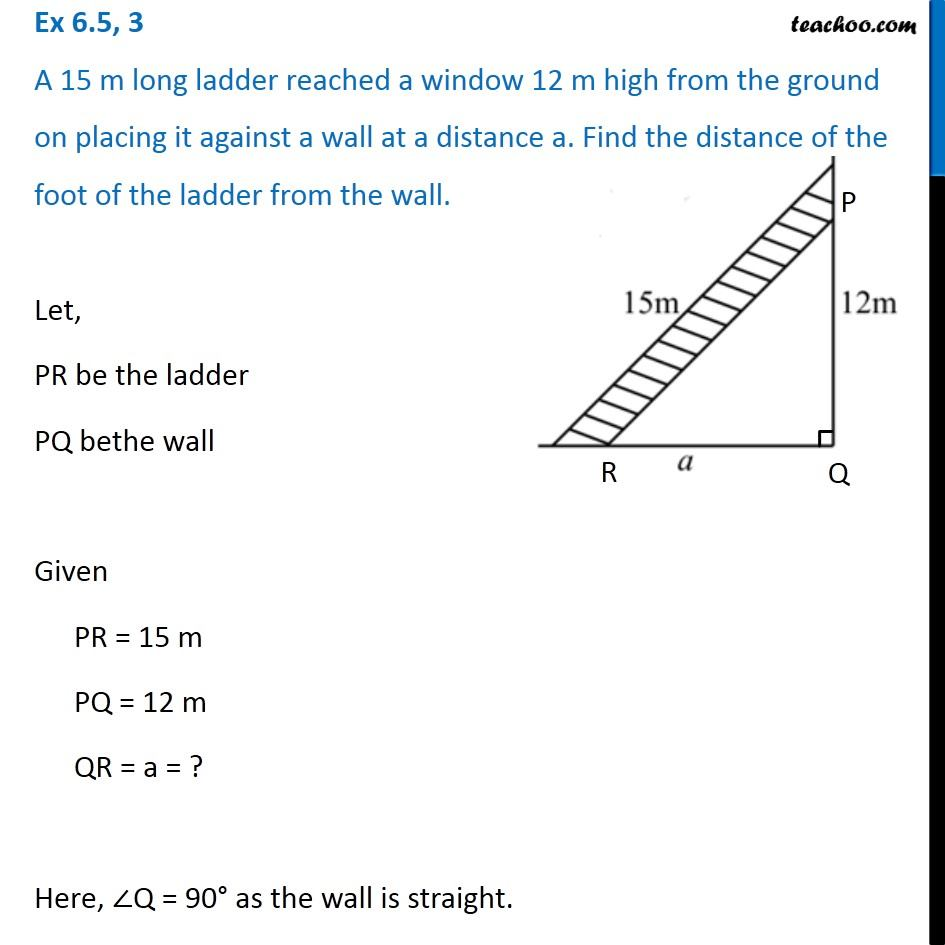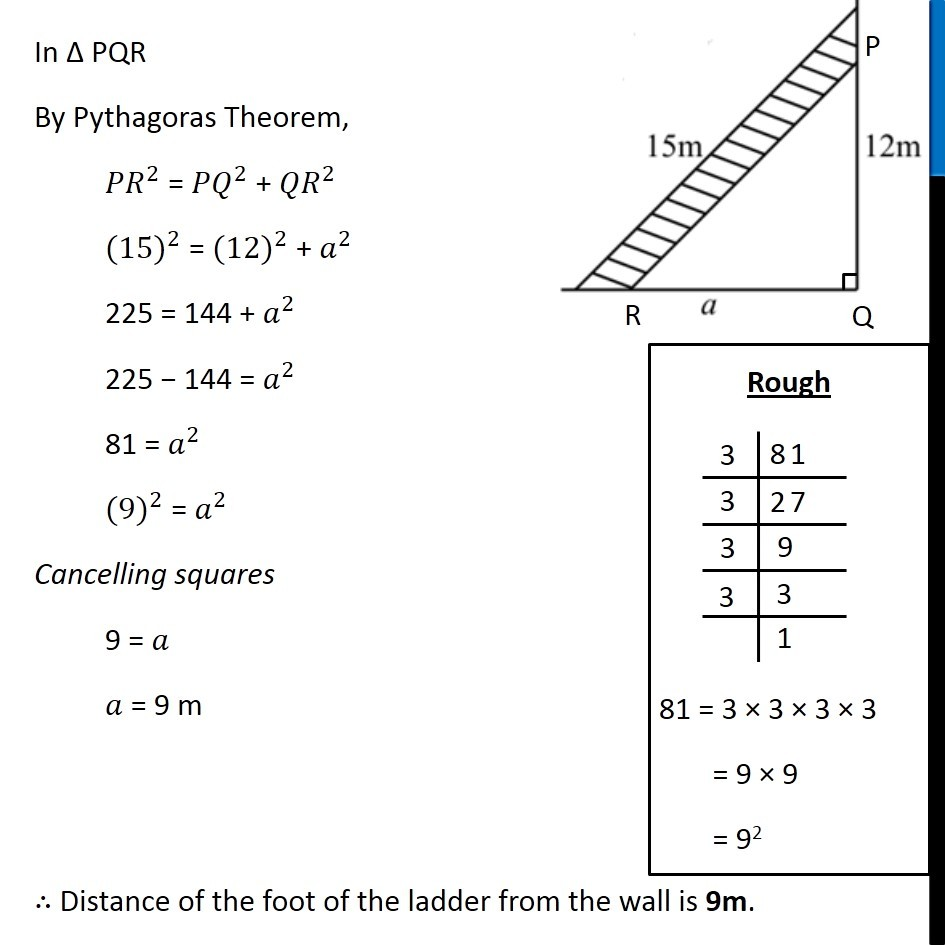1. Chapter 6 Class 7 Triangle and its Properties
2. Serial order wise
3. Ex 6.5

Transcript

Ex 6.5, 3 A 15 m long ladder reached a window 12 m high from the ground on placing it against a wall at a distance a. Find the distance of the foot of the ladder from the wall.Let, PR be the ladder PQ bethe wall Given PR = 15 m PQ = 12 m QR = a = ? Here, ∠Q = 90° as the wall is straight. In Δ PQR By Pythagoras Theorem, 〖𝑃𝑅〗^2 = 〖𝑃𝑄〗^2 + 〖𝑄𝑅〗^2 〖(15)〗^2 = 〖(12)〗^2 + 𝑎^2 225 = 144 + 𝑎^2 225 − 144 = 𝑎^2 81 = 𝑎^2 〖(9)〗^2 = 𝑎^2 Cancelling squares 9 = 𝑎 𝑎 = 9 m ∴ Distance of the foot of the ladder from the wall is 9m.